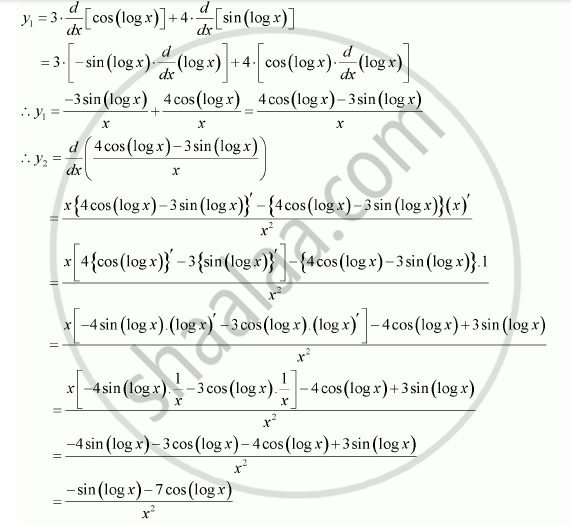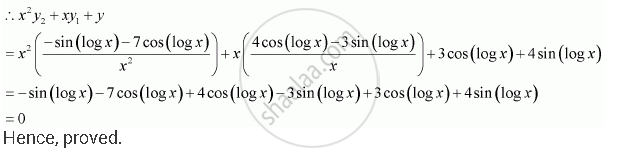Share

# If Y = 3 Cos (Log X) + 4 Sin (Log X), Show that X2 Y2 + Xy1 + Y = 0 - CBSE (Commerce) Class 12 - Mathematics

#### Question

If y = 3 cos (log x) + 4 sin (log x), show that x2 y2 + xy1 + y = 0

#### Solution

It is given that ,y = 3 cos (log x) + 4 sin (log x),

Then,Is there an error in this question or solution?

#### APPEARS IN

NCERT Solution for Mathematics Textbook for Class 12 (2018 to Current)
Chapter 5: Continuity and Differentiability
Q: 13 | Page no. 184

#### Video TutorialsVIEW ALL 

Solution If Y = 3 Cos (Log X) + 4 Sin (Log X), Show that X2 Y2 + Xy1 + Y = 0 Concept: Second Order Derivative.
S